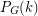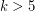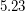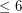# Real roots of the flow polynomial

 Importance: Medium ✭✭
 Author(s): Welsh, Dominic J. A.
 Subject: Graph Theory » Coloring » » Nowhere-zero flows
 Keywords: flow polynomial nowhere-zero flow
 Posted by: mdevos on: March 7th, 2007
Conjecture   All real roots of nonzero flow polynomials are at most 4.

For every graph, letbe the chromatic polynomial ofand letbe the flow polynomial of. Ifis loopless, thenfor all sufficiently large integers(as= # of k-colorings of). It follows from Seymour's 6-flow theorem that ifhas no bridge, thenfor all integers(as= # of nowhere-zero flows in the group of integers modulo). It is natural to ask if all real roots of these polynomials are small. For the chromatic polynomial,, this is not the case. There exist graphs with chromatic number 3 for whichhas arbitrarily large real roots. The above conjecture asserts that the flow polynomial exhibits the opposite behavior. One word of caution, it is known that the set of roots of flow polynomials is dense in the complex plane.

## Bibliography

[S] P.D. Seymour, Nowhere-Zero 6-Flows, J. Combinatorial Theory Ser. B 30 (1981) 130-135. MathSciNet

* indicates original appearance(s) of problem.

### Not bounded by 5, either

A preprint by Jesper L. Jacobsen and Jesus Salas claims that there are graphs with roots of their flow polynomial being above 5. The generalized Petersen graphs G(7n,7) are claimed to have roots of flow polynomial that accumulate at approximately.

I suppose this makes the original conjecture truly false. An interesting variant, though, is to find out, if all roots of flow polynomials are. (Thanks to Bojan Mohar for pointing out the paper to me.)

### Welsh's conjecture is false

Welsh's conjecture on flow roots is false. In fact, many cubic graphs with reasonably large girth and enough vertices have flow roots between 4 and 5, and it is almost certain that we can find graphs with flow roots arbitrarily close to 5.

However I strongly believe that "All real roots of nonzero flow polynomials are at most FIVE".

See my recent survey article "Recent results on chromatic and flow roots of graphs and matroids, Surveys in Combinatorics 2009" for more detail.

Gordon Royle